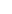Let’s suppose we want to invest and in order to do this buy the coin A, which belongs to the category of POW coins. In particular:

Let’s suppose we buy 1500 coins at the price of 0.00007450 BTC.
And let's say that our goal is to sell them after a while at the price of 0.00007850 BTC.
Therefore, the desired total gross profit from this investment will be:
1500x (0.00007850 -0.00007450) = 1500 x 0.00000400 = 0.00600000 BTC.

Let's also assume that despite our expectations, the price of coin A drops to the price of 0.00007150BTC, and the odds for an increase are considered to be minimized.
One out of several different strategies for dealing with such situations is:

• We hold 1000 pieces of coin A and we calculate the desired total revenue from the sale of 500 coins, which is: 500 x 0.00007850 = 0.03925000BTC.

• We sell the 500 coins at the current available price. So we have: 500 x 0.00007150 = 0.03575000BTC.

• With the amount of 0.03575000 we buy a B coin, which should belong to the POS coin category. For example, let’s suppose that the price at which we can buy coin B is 0.00125000 BTC. So, we buy 0.03575000 / 0.00125000 = 28.6 coins.

• We calculate at what price the currency B should be sold to achieve the total desired profit: 0.03925000 / 28.6 = 0.00137238.

• One option is to place it in a sell order and wait for some price increase, but that could be done in any other coin, POS or POW. What gives us another advantage is that we can send it to a wallet or a platform that rewards us with Staking Rewards. With the price fixed at 0.00125000, we will need: 0.03925000 / 0.00125000 = 31.4 currencies in total, i.e. 2.8 extra currencies (31.4 -28.6) from Staking Rewards. It goes without saying that if the current price of B is changed, the number of coins we need from Staking Rewards changes too.

• If this strategy is successful, we can continue with the remaining unsold coins A.to signal pagePOS coins as risk spreading instruments ❺❺❺ - average rating 5 from 5 (based on 204 user reviews)